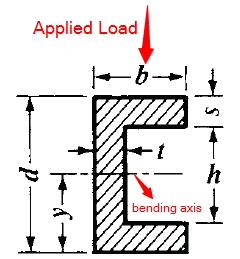This is the C Beam Moment of Inertia Calculator. Simply enter the dimensions below for C beam to find the moment of inertia.d = h = b = t =